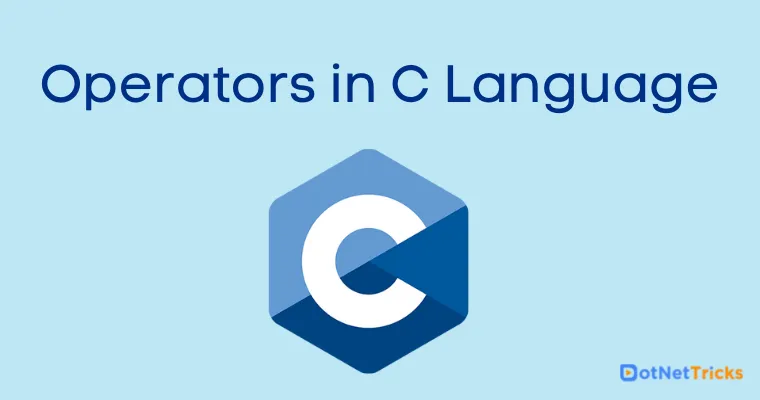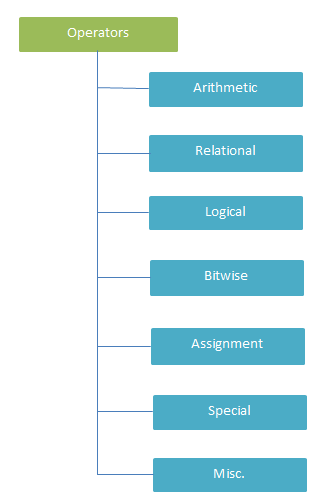# Operators in C Language

21 Aug 2022
Intermediate
66.6K Views

C language supports a rich set of built-in operators. An operator is a special symbol that tells the compiler to perform specific mathematical or logical operations. Operators in programming languages are taken from mathematics.

## Types of Operators

1. Arithmetic operators

2. Relational operators

3. Logical operators

4. Bitwise operators

5. Assignment operators

6. Type Information Operators(Special operators)## Arithmetic Operators

An operator is a special symbol that tells the compiler to perform specific mathematical or logical operations. Operators in programming languages are taken from mathematics.

Operators
Name
C Example
+
X + Y will give 7
-
Substraction
X - Y will give 3
*
Multimlication
X * Y will give 10
/
Divition
X / Y will give 2
%
Modulus
X % Y will give 1
++
Preincrement and Postincrement
X = ++Y, X = Y++ will give 3, 2
--
Predecrement and Postdecrement
X = --Y, X = Y-- will give 4, 2

### Arithmetic Operators Example

```#include <stdio.h>
#include <conio.h>
void main()
{
int a b ,c;
clrscr(); // Clear the screen
a=10;
b=3;
c = a+b;
printf("Total (a+b) = %d \n",c);

c = a-b;
printf("Sub (a-b )= %d \n",c);

c = a*b;
printf("Mul (a*b) = %d \n",c);

c=a/b;
printf("Div (a/b) = %d \n",c);

c=a%b;
printf("Remainder (a Mod b) = %d \n",c);

getch();
}
```
Output
```Total (a+b) = 13
Sub (a-b ) = 7
Mul (a*b) = 30
Div (a/b) = 3
Remainder (a Mod b) = 1
```

## Relational operators

These operators are used to compare values and always result in boolean value (True or False). The following is a table of relational operators in C. Suppose you have two integer variables X, Y and having values 5, 2 respectively then

Operators
Name
C Example
>
Greater than
X > Y will give True
<
Less than
X < Y will give False
>=
Greaterthan or Equalto
X >= Y will give True
<=
Lessthan or Equalto
X <= Y will give False
>==
Equalto
X == Y will give False
!=
NotEqualto
X != Y will give True

### Relational Operators Example

```#include <stdio.h>
#include <conio.h>
void main()
{
int a = 10, b = 20, c = 30;
clrscr();
printf("%d == %d result %d \n", a, b, a == b);
printf("%d == %d result %d \n", a, c, a == c);
printf("%d > %d result %d \n", a, b, a > b);
printf("%d > %d result %d \n", a, c, a > c);
printf("%d < %d result %d \n", a, b, a < b);
printf("%d < %d result %d \n", a, c, a < c);
printf("%d != %d result %d \n", a, b, a != b);
printf("%d != %d result %d \n", a, c, a != c);
printf("%d >= %d result %d \n", a, b, a >= b);
printf("%d >= %d result %d \n", a, c, a >= c);
printf("%d <= %d result %d \n", a, b, a <= b);
printf("%d <= %d result %d \n", a, c, a <= c);
getch();
}
```
Output
```10 == 30 result 0
10 > 20 result 0
10 > 30 result 0
10 < 20 result 1
10 < 30 result 1
10 != 20 result 1
10 != 30 result 1
10 >= 20 result 0
10 >= 30 result 0
10 <= 20 result 1
10 <= 30 result 1
```

## Logical Operators

These operators are used to compare values and always result in boolean value (True or False). The following is a table of logical operators in C. Suppose you have two boolean variables X, Y and having values True, False respectively then

These operators are used to perform logical operations on the given two variables.

Operators
Name
C Example
&&
Logical AND
X && Y will give False
||
Logical OR
X || Y will give True
>!
Logical NOT
!(X), !(Y) will give False, True

### Logical Operators Example

```#include <stdio.h>
#include <conio.h>
void main()
{
int a = 30,b =10, c=10;
clrscr();
if(a>b && a>c )
printf("\n a is grather then b and c ");
else
printf("\n a is not grather then b and c ");
getch();
}
```
Output
```a is grather then b and c
```

## Bitwise Operators

These operators work on bits of a binary number and perform bit by bit operation. The following is a table of bitwise operators in C. Suppose you have two integer variables X, Y and having values 4, 5 respectively and theirs binary equivalent would be 100, 101 then

Operators
Name
C Example
|
Bitwise OR
X | Y will give 5 i.e. 101
&
Bitwise AND
X & Y will give 4 i.e. 100
~
Bitwise NOT
~(X), ~(Y) will give -5, -6 i.e. -101, -110
^
Bitwise Exclusive OR
X^Y will give 1 i.e. 1

### Bitwise Operators Example

```#include <stdio.h>
#include <conio.h>
void main()
{
int m = 10,n = 20,AND,OR,XOR,NOT ;
clrscr();
AND = (m&n);
OR = (m|n);
NOT = (~m);
XOR = (m^n);
printf("AND value = %d\n",AND );
printf("OR value = %d\n",OR );
printf("NOT value = %d\n",NOT );
printf("XOR value = %d\n",XOR );
printf("left shift value = %d\n", m << 1);
printf("right shift value = %d\n", m >> 1);
getch();
}
```
Output
```AND value =0
OR value = 30
NOT value = -11
XOR value = 30
left shift value = 20
right shift value = 5
```

## Assignment Operators

These operators are used to assign a new value to a variable, a property, an event, or an indexer element. The following is a table of assignments operators in C. Suppose you have two integer variables X, Y and having values 5, 2 then

Operators
Name
C Example
=
Equalto
X = Y will give 2
+=
Plus Equalto
X += Y will give 7
-=
Minus Equalto
X -= Y will give 3
*=
Multiply Equalto
X *= Y will give 2
/=
Divide Equato
X /= Y will give 2
%=
modulus Equalto
X %= Y will give 1

### Assignment Operators Example

```#include <stdio.h>
#include <conio.h>
void main()
{
int a ,b;
clrscr();
a=10;
b = a;
printf("b = %d \n", b);

b += a; // or b = b+a
printf("b = %d \n", b);

b -= a; // or b = b-a
printf("b = %d \n", b);

b *= a; // or b = b*a
printf("b = %d \n", b);

b /= a; // or b = b/a
printf("b = %d \n", b);

b %= a; // or b = b%a
printf("b = %d \n", b);
getch();
}
```
Output
```b = 10
b = 20
b = 10
b = 100
b = 10
b = 0
```

## Type Information Operators (Special operators)

These operators are used to provides information about a particular type. The following is a table of type information operators in C.

Operators
Description
Example
sizeof()
Returns the size of a value type.
sizeof(int) will give 2

### Sizeof Operator Example

```#include <stdio.h>
#include <conio.h>
void main()
{
int a;
char b;
float c;
double d;
clrscr();
printf("Storage size of data type int is :%d \n",sizeof(a));
printf("Storage size of data type char is :%d \n",sizeof(b));
printf("Storage size of data type float is :%d \n",sizeof(c));
printf("Storage size of data type double is :%d\n",sizeof(d));
getch();
}
```

## Misc. Operators

There are some more important operators supported by C. The following is a table of some Misc operators in C. Suppose you have two integer variables X, Y and having values 5, 2 then

Operators
Name
C Example
?:
Conditional Expression/Ternary Operator
X > Y ? X : Y will give 5
*
Pointer to a variable
*X will pointer to X variable.
&
Returns memory address of a variable
X > &X will give address of XY ? X : Y will give 5

### Misc. Operators Example

```#include <stdio.h>
#include <conio.h>
void main()
{
int a = 10,b = 20 ,m ;
clrscr();
m= a>b? a+b: a+10;
printf("Result = %d\n",m);
getch();
}
```
Output
```Result = 20
```
```#include <stdio.h>
#include <conio.h>
void main()
{
int *ptr, q;
q = 10;
ptr = &q;
printf("%d", *ptr);
getch();
}
```
Output
```Storage size of data type int is :4
Storage size of data type char is :1
Storage size of data type float is :4
Storage size of data type double is :8
```